# University Projects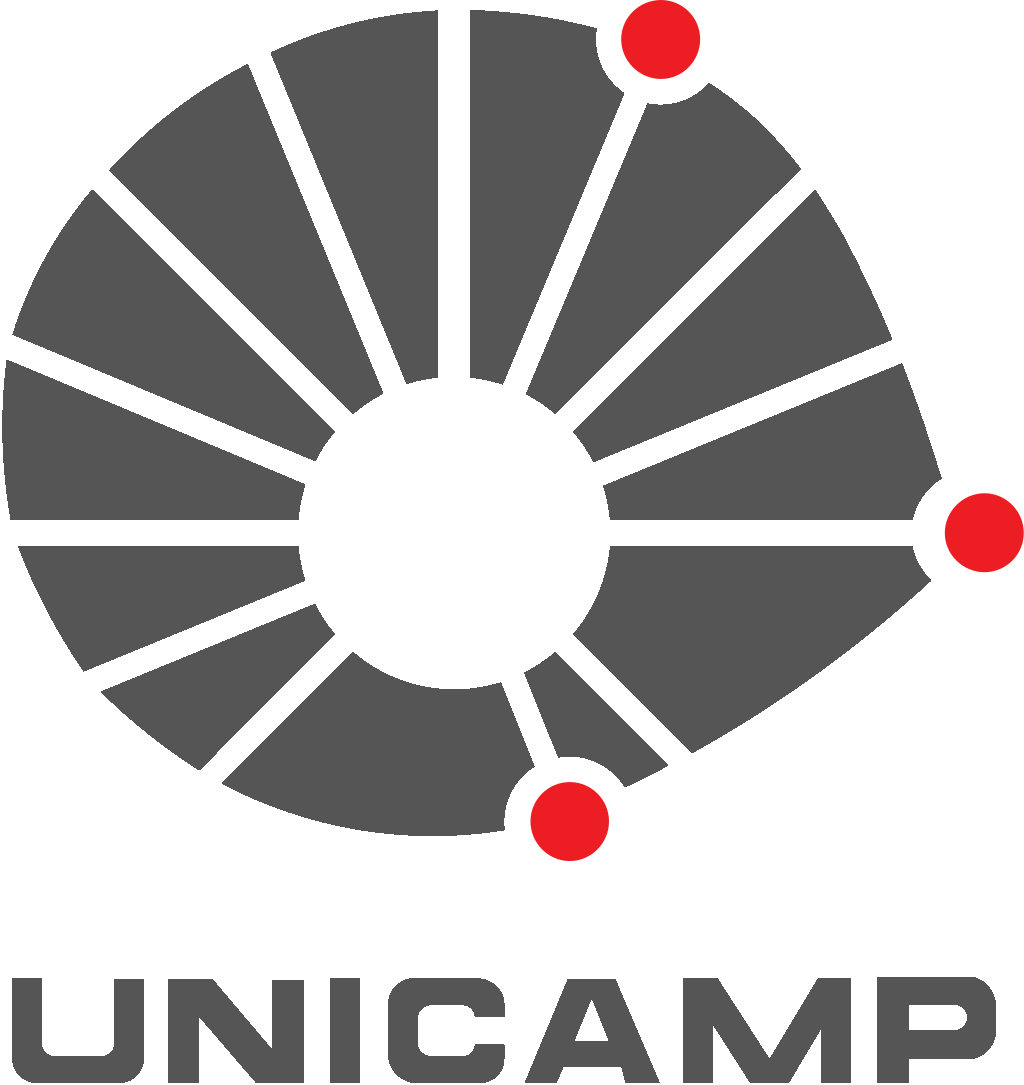Here I share projects that I have done for some subjects in the computer science course.

## Artificial Intelligence (2019)

Used languages: Python and C#

This course was taken in the first semestre of 2019 at Unicamp. You can find the complete project in this repository. Introductory study of the fundamentals and applications of Artificial Intelligence. History and principles of AI. Problem solving. Knowledge representation. Applications. The following topics have been covered:

• AI history and principles
• Smart agents
• Search without information, search with information and competitive search.
• Genetic algorithms
• Constraint satisfaction problem
• Evolutionary computing
• Planning
• Fuzzy systems
• Uncertainty and Bayesian Networks
• Machine learning
• Learning models (supervised, unsupervised and semi-supervised)
• Decision trees
• Neural networks
• Markov Models and Reinforcement Learning
• AI topics

As a complement to the course, we had to implement 4 projects that are described below.

##### Project 1 - Graph Search Algorithms

There are several algorithms that can be used to automate the process of finding a minimum path between two points. Thus, this work aims to compare some of these possibilities (Breadth First Search, Uniform Cost Search, A * and Greedy) using different heuristics through maps generated randomly where a point A (red) must reach a point B (blue). You can find the codes used in this project here.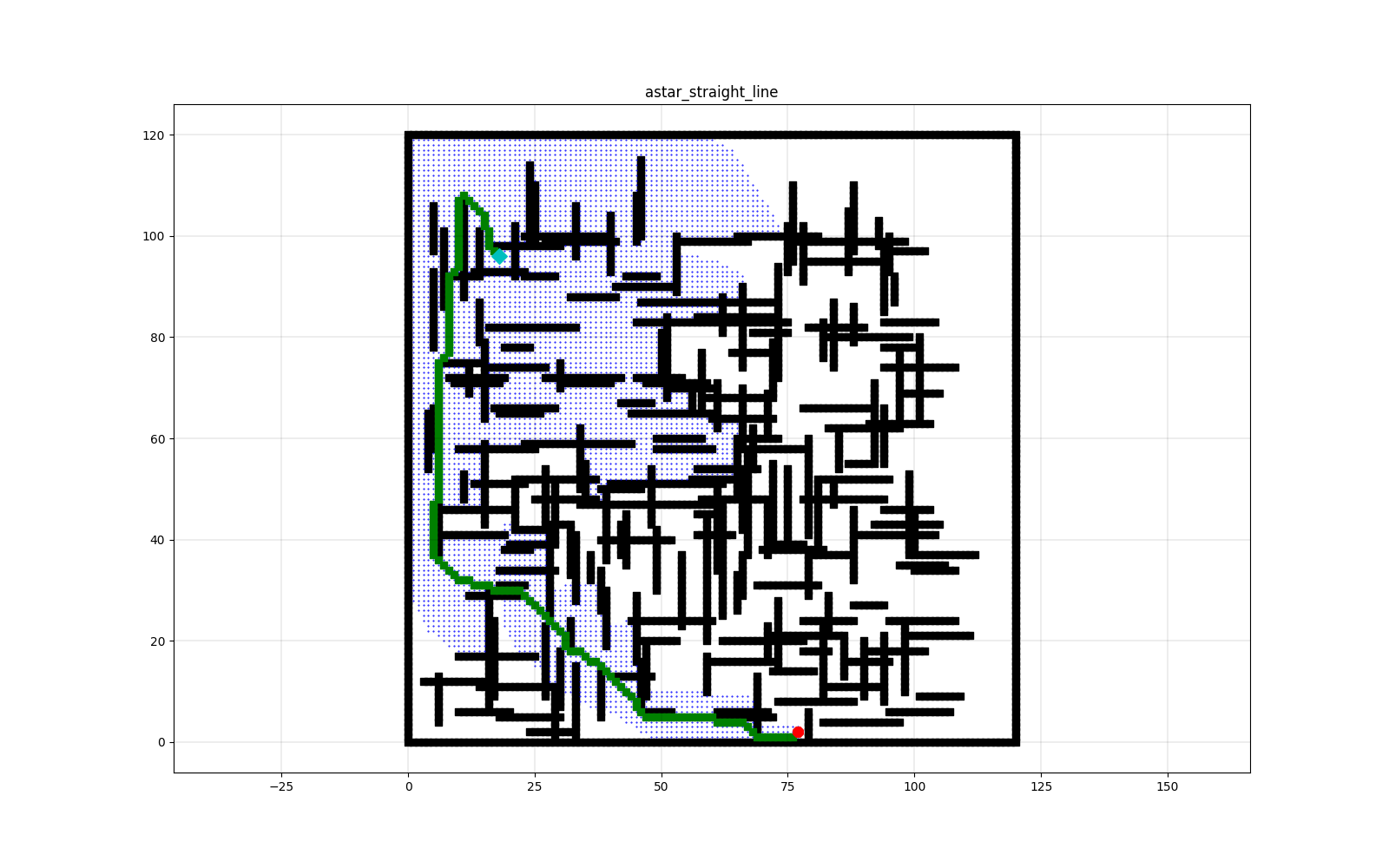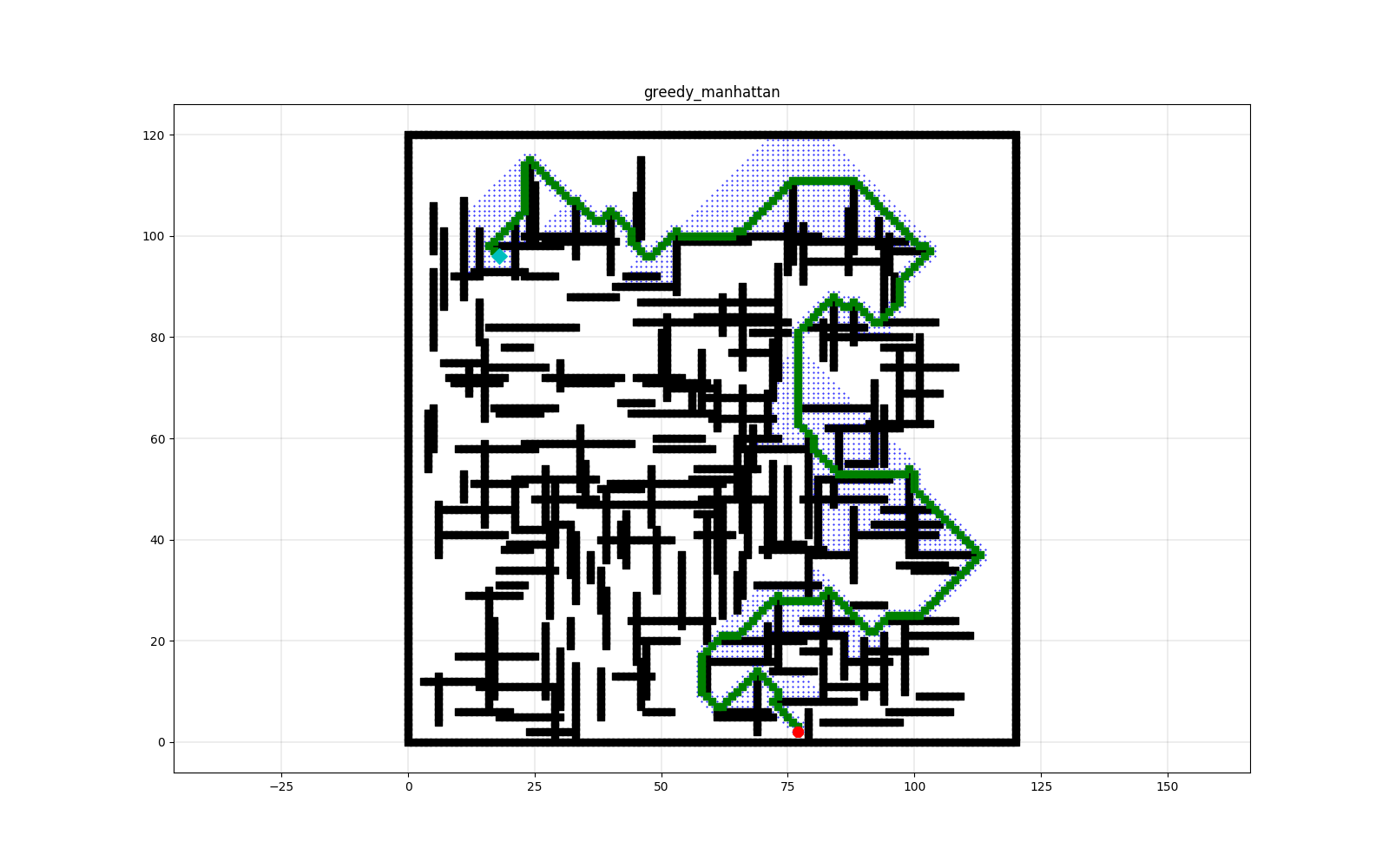##### Project 2 - Genetic Algorithms in Optimization of RFID Antenna Readings

For RFID antennas to work properly, the parameter settings of the modules must be well selected. However, given the nature of the problem, this is not feasible to do by testing all possibilities. In the search for brute force, an algorithm with an order of complexity O(101n) would be necessary, with n = 31, in the simulation used in this project. Thus, genetic algorithms were used in search of the ideal configuration result. Different parameters were used in the algorithm, producing different solutions. The results obtained solve the problem in different ways and are, in general, quite satisfactory. Our best algorithm achieved the ideal result in just 21s:38ms. You can find the codes used in this project here.

##### Project 3 - Control Algorithm for Autonomous Robot Using Fuzzy Systems

Robots, sensors and scenarios were simulated using the V-REP software. Different scenarios were created so that the robot could achieve objectives by avoiding obstacles. You can find the codes used in this project here.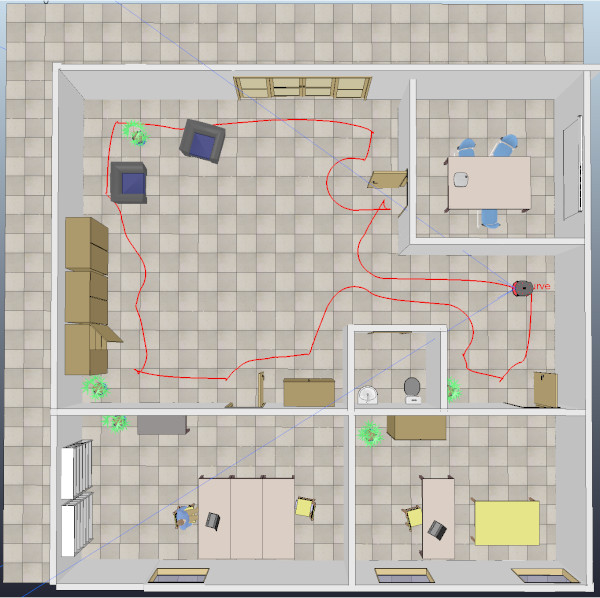##### Project 4 - Face Recognition

This work addresses the problem of face recognition in images through a regression tree algorithm using landmarks. This project shows how the model was trained and evaluated. It also shows some practical applications.

Given a single photo and an associated name, the algorithm is able to recognize that person in other photos or videos, even in real time. You can find the codes used in this project here.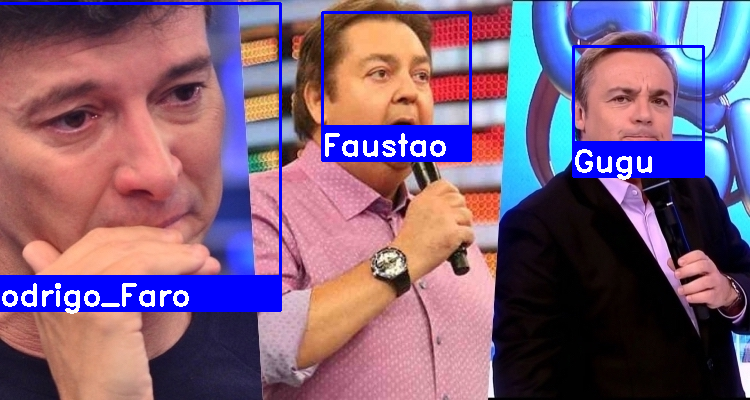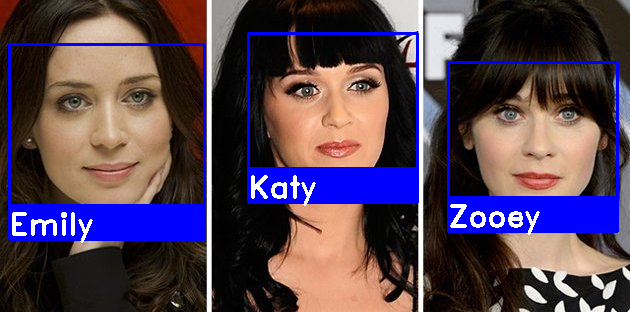## Introduction to Digital Image Processing (2019)

Used language: Python

This course was taken in the first semester of 2019 at Unicamp. You can find the complete project in this repository. objectives: to present theoretical and practical aspects related to the image processing area. Describe techniques for image acquisition, transformation and analysis using a computer. The following topics have been covered:

• Introduction
• Representation of digital images
• Componentes of image processing system
• Application areas
• Fundamentals of Digital Images
• Human visual system
• Image formation
• Sampling and quantization
• Spatial resolution and image depth Basic pixel relationships (neighborhood, connectivity, adjacency, path, distance measurements, related components)
• Noise in images
• Image Enhancement Techniques
• Image quality
• Gray scale transformation
• Histogram of images
• Correlation and convolution operations
• Spatial domain filtering
• Frequency domain filtering
• Image Segmentation
• Discontinuity detection
• Edge detection
• Threshold (global and local)
• Region-based segmentation
• Representation and Description
• Representation schemes (chain code, polygonal approximations, signatures, skeleton of a region)
• Descriptors (basic descriptors, Fourier descriptors, moments, regional descriptors, texture)
• Mathematical Morphology
• Image Compression
• Fundamentals of image compression (coding redundancy, interpixel redundancy, psychovisual redundancy)
• Lossless compression
• Lossy compression
• Image Registration
• Geometric transformations
• Spatial transformations
• Image interpolation
• Correspondence between images
• Image Classification (TRABALHO 5)
• Image analysis elements
• Patterns and pattern classes
• Decision methods (marriage, statistical classifiers, neural networks, fuzzy logic)

Projects: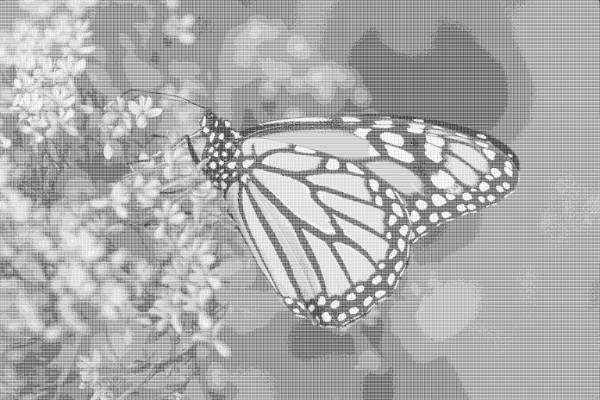Project 2 - This image was produced using only two colors (black and white), with no other shades of gray

## Databases (2018)

Used language: MySQL

This course was taken in the first semester of 2018 at Unicamp. You can find the complete project in this repository. The following topics have been covered:

• Introduction - database management architecture
• Data models: introduction to the concepts of data modeling, conceptual and logical models, including relational models and normalization
• Application design based on conceptual models
• The relational model: definitions and formalization
• Mapping the ER model to the relational model; mapping between models, from conceptual to physical
• Processing of queries in relational algebra
• Query optimization
• Transaction processing - Protection, concurrency control and recovery
• Non-strictly relational database systems
• Development of practical projects

Projects: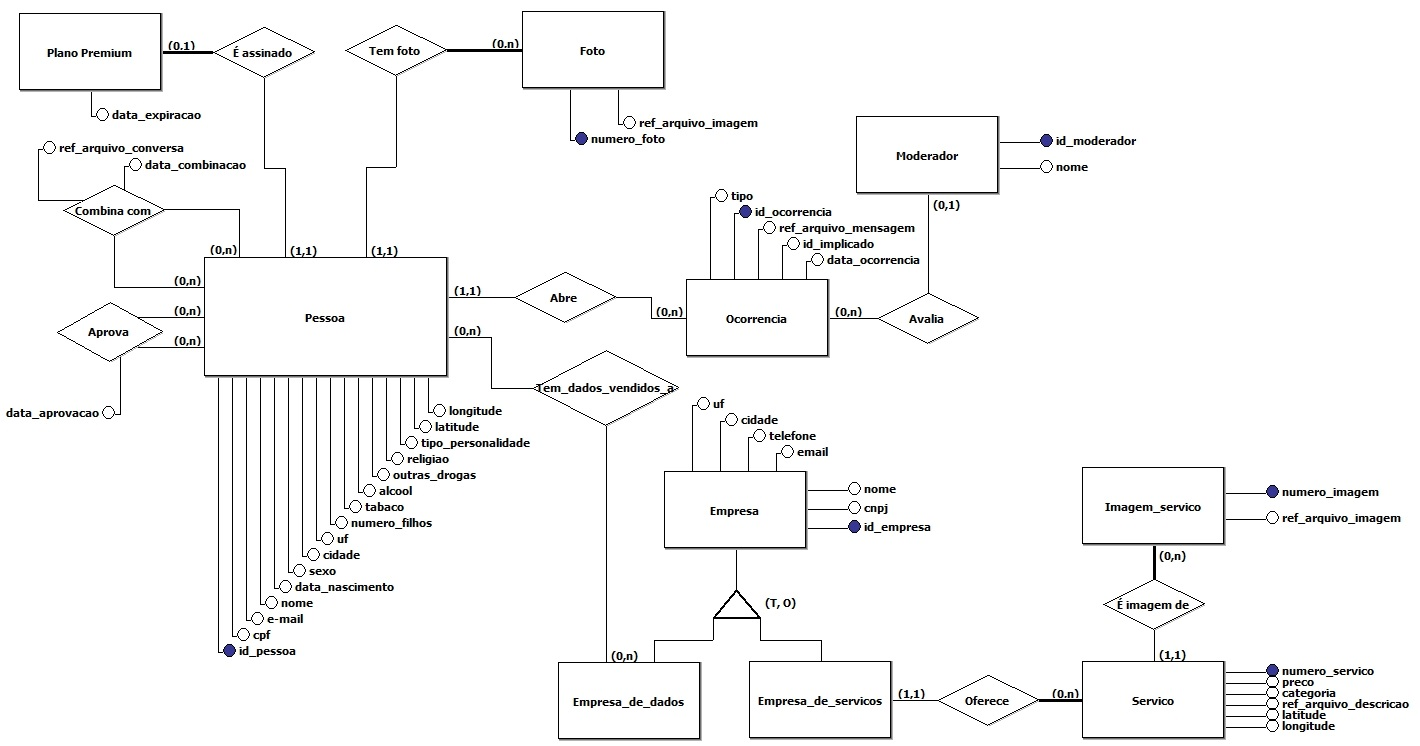Entity–relationship model

## Software Engineering (2018)

Used languages: UML and Java

This course was taken in the first semester of 2018 at Unicamp. The following topics have been covered:

• Software Engineering Paradigms
• Agile Methodologies
• Software Processes
• Software Process Models
• Requirements Extraction and Specification
• Analysis and Design of Software Systems
• Architecture Patterns and Design Patterns

Although this is a design-oriented discipline, a system has been implemented in the past few weeks using java and can be found here.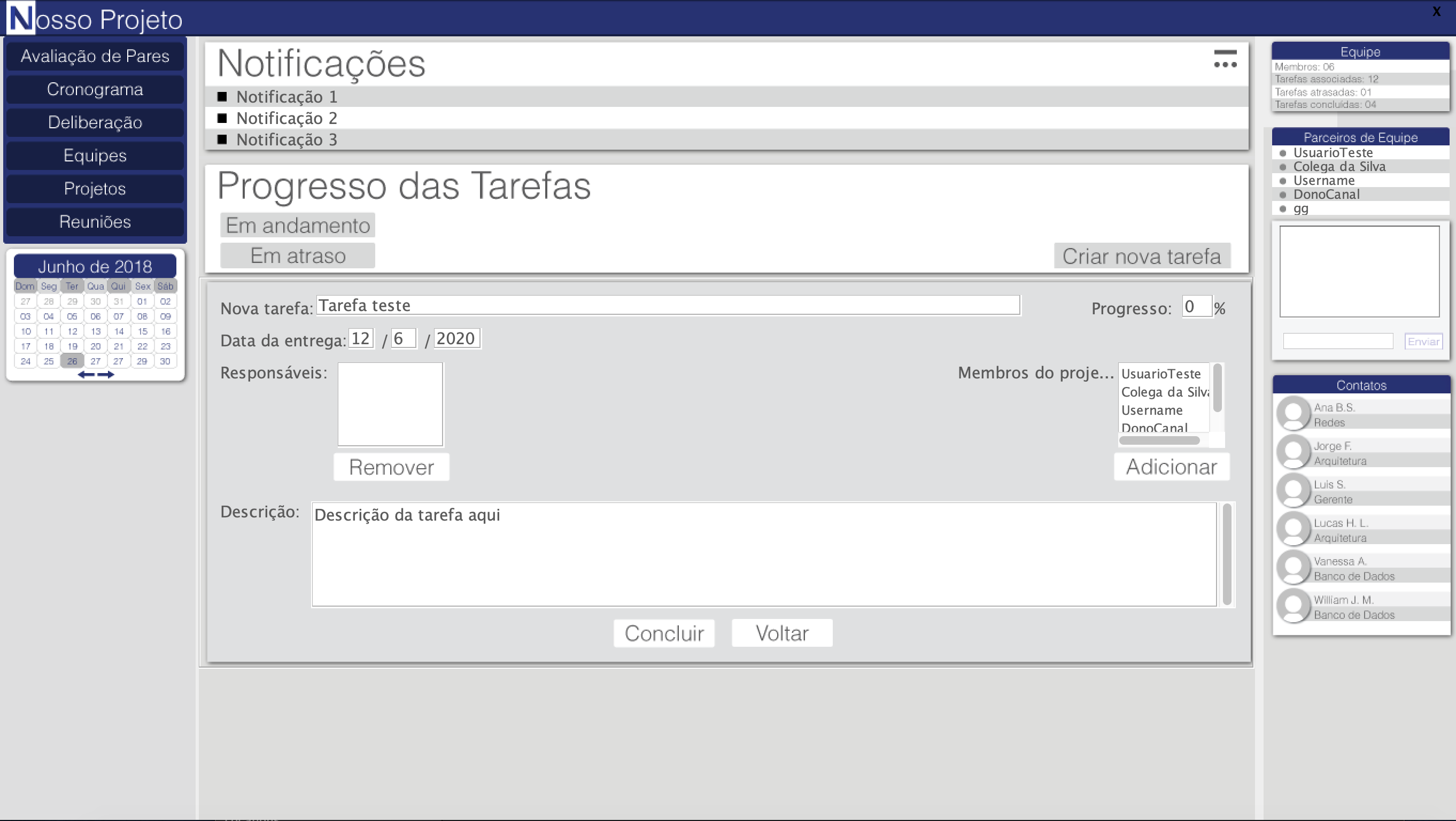## Computer Organization and Assembly (2018)

Used languages: Assembly(ARM and LEG)

This course was taken in the first semester of 2018 at Unicamp. You can find the complete project in this repository. The following topics have been covered:

• Computer history
• Basic computer organization
• Memory and addressing. Representation of information in memory
• Introduction to processor architecture
• Set of instructions: access to memory, arithmetic, logic and shift operations, procedures and functions
• Assembly Language Programming, using two sets of instructions: LEG and ARM processors
• Relationship with high-level languages, rudiments of compilation: implementation of efficient repetitions, data structures, passing parameters, function return values, object orientation
• Input / Output instructions, interruptions and access to peripherals
• Assemblers, connectors and loaders

Projects:

• Several tasks have been programmed in the ARM and LEG languages and can be found here.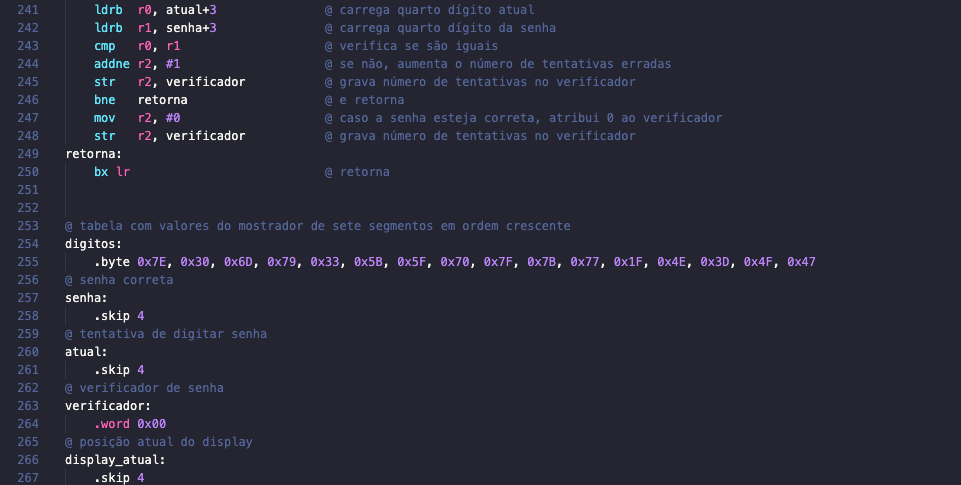ARM code

## Design and Analysis of Algorithms (2017)

Used language: C++

This course was taken in the second semester of 2017 at Unicamp. objectives: To analyze the correctness and complexity of algorithms in a formal way. Model common problems and subproblems. Design and develop algorithms using classical techniques. The following topics have been covered:

• Introduction
• Algorithm complexity
• Correction of algorithms
• Design of algorithms by induction and divide-and-conquer
• Sorting algorithms
• Introduction to randomized algorithms and ordering by partitioning
• Linear time ordering
• Order statistics
• Dynamic programming
• Greedy algorithms

Although this course is much more theoretical and mathematical, some practical projects have been implemented in C ++ and can be found here.

## Data Structures (2016)

Used languages: C

The following topics have been covered:

• Basic data representation structures
• Abstract Data Type
• contents: dynamic memory allocation; use of pointing variables and vectors; abstract data type definition
• objectives: to allocate data dynamically; abstract complex structures by separating data, operations and implementation
• Connected Structures, Notions of Efficiency
• contents: node; asymptotic notation
• objectives: to create lists dynamically; calculate the efficiency of simple algorithms
• List operations and variations
• contents: circular lists, double linked lists, operations
• objectives: to implement operations and list variants
• Stacks and Queues
• contents: dynamic set; collection removal policies; stacks application
• objectives: to identify real situations that can be modeled as stacks and queues
• Recursive programming techniques
• Recursion
• contents: simple recursion; efficiency of recursive algorithms; call stack
• objectives: simulate recursion using call stack
• Divide-and-conquer
• contents: recursive sorting algorithms: merge-sort and quick-sort
• objectives: to solve subproblems recursively using divide-and-conquer
• Backtracking
• contents: N-queens problem; partial problem solution; backtracking troubleshooting
• objectives: to identify subproblems; solve subproblems recursively using backtracking
• Dynamic sets on trees
• Trees
• contents: binary tree
• objectives: represent structures and sets hierarchically
• Binary Search Trees
• contents: search, insertion and removal in binary search trees
• objectives: to implement dynamic sets in a binary tree
• Balanced Trees
• contents: AVL tree
• objectives: to implement dynamic sets in a binary tree efficiently
• Splay Tree
• contents: splay tree; principle of locality and reference
• objectives: to identify situations in which the principle of locality and reference can be used to improve the efficiency of a program
• B-Tree
• contents: B-tree; disk page and latency concepts
• objectives: to implement dynamic assembly in permanent storage medium
• Priority queues and heap-sort
• contents: max-heap; heap-sort ordering method
• objectives: to implement operation with maximum efficiency
• Prefix Tree and Huffman Encoding
• contents: dictionary; coding; Huffman’s algorithm
• objectives: apply priority queue; get Huffman coding
• Evaluation of data structures for dynamic sets
• contents: overview of trees
• objectives: evaluate the best algorithm and data structure for problems with dynamic data set
• Advanced data structures
• Hashing
• contents: hashing function, hash table
• objectives: to implement dynamic set in almost constant time
• Graphs
• contents: definition of graphs; graph terminology
• objectives: to identify situations that can be modeled with graphs
• Graph representation
• contents: representation of graphs in memory: adjacency list and adjacency matrix; depth first search and breadth first search
• objectives: to implement basic operations with graphs
• Graph algorithms
• contents: shortest path algorithm (Dijkstra); topological sorting
• objectives: apply classic graph algorithms to real problems
• Generalized lists and general tree
• contents: generalized lists: atom, list; general tree
• objectives: to interpret data structures as generalized lists
• Memory management
• contents: free list; peer system; garbage collection; reference count
• objectives: to understand dynamic allocation implementations and their interference with data structure

Some projects can be found here.